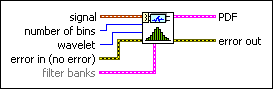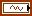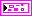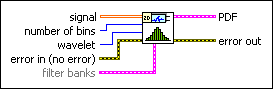# WA Probability Density Function Estimation VI

LabVIEW 2014 Advanced Signal Processing Toolkit Help

Edition Date: June 2014

Part Number: 372656C-01

»View Product InfoDownload Help (Windows Only)

Owning Palette: Feature Extraction VIs

Estimates the probability density function (PDF) of 1D or 2D signals from the error-reduced statistical histogram. Wire data to the signal input to determine the polymorphic instance to use or manually select the instance.

Use the pull-down menu to select an instance of this VI.

 Select an instance WA Probability Density Function Estimation (Waveform)WA Probability Density Function Estimation (1D Array)WA Probability Density Function Estimation (2D Array)

## WA Probability Density Function Estimation (Waveform)signal specifies the input signal.number of bins specifies the number of bins to use to estimate the statistical histogram of signal.wavelet specifies the wavelet type to use for the discrete wavelet analysis. The default is db02. The options include two types: orthogonal (Haar, Daubechies (dbxx), Coiflets (coifx), Symmlets (symx)) and biorthogonal (Biorthogonal (biorx_x), including FBI (bior4_4 (FBI))), where x indicates the order of the wavelet. The higher the order, the smoother the wavelet. The orthogonal wavelets are not redundant and are suitable for signal or image denoising and compression. The biorthogonal wavelets usually have the linear phase property and are suitable for signal or image feature extraction. If you want to use other types of wavelets, do not wire this input. Instead, use the Wavelet Design Express VI to design the wavelet you want, bundle the resulting analysis and synthesis filters, and then wire them to the filter banks input.error in describes error conditions that occur before this node runs. This input provides standard error in functionality.filter banks specifies the analysis filter banks and the synthesis filter banks for the wavelet you specify. If you specify a value for filter banks, this VI ignores the settings in the wavelet input. You can use the Wavelet Design Express VI to design the analysis filters and the corresponding synthesis filters.analysis filters specifies the coefficients of the lowpass analysis filters and the highpass analysis filters for the wavelet you specify.lowpass specifies the coefficients of the lowpass analysis filter, which this VI uses to compute the approximation coefficients.highpass specifies the coefficients of the highpass analysis filter, which this VI uses to compute the detail coefficients.synthesis filters specifies the coefficients of the lowpass synthesis filters and the highpass synthesis filters for the wavelet you specify.lowpass specifies the coefficients of the lowpass synthesis filter, which this VI uses to filter the interpolated approximation coefficients in the wavelet reconstruction.highpass specifies the coefficients of the highpass synthesis filter, which this VI uses to filter the interpolated detail coefficients in the wavelet reconstruction.PDF returns the estimated probability density function of signal on an XY graph.error out contains error information. This output provides standard error out functionality.

## WA Probability Density Function Estimation (1D Array)signal specifies the input signal.number of bins specifies the number of bins to use to estimate the statistical histogram of signal.wavelet specifies the wavelet type to use for the discrete wavelet analysis. The default is db02. The options include two types: orthogonal (Haar, Daubechies (dbxx), Coiflets (coifx), Symmlets (symx)) and biorthogonal (Biorthogonal (biorx_x), including FBI (bior4_4 (FBI))), where x indicates the order of the wavelet. The higher the order, the smoother the wavelet. The orthogonal wavelets are not redundant and are suitable for signal or image denoising and compression. The biorthogonal wavelets usually have the linear phase property and are suitable for signal or image feature extraction. If you want to use other types of wavelets, do not wire this input. Instead, use the Wavelet Design Express VI to design the wavelet you want, bundle the resulting analysis and synthesis filters, and then wire them to the filter banks input.error in describes error conditions that occur before this node runs. This input provides standard error in functionality.filter banks specifies the analysis filter banks and the synthesis filter banks for the wavelet you specify. If you specify a value for filter banks, this VI ignores the settings in the wavelet input. You can use the Wavelet Design Express VI to design the analysis filters and the corresponding synthesis filters.analysis filters specifies the coefficients of the lowpass analysis filters and the highpass analysis filters for the wavelet you specify.lowpass specifies the coefficients of the lowpass analysis filter, which this VI uses to compute the approximation coefficients.highpass specifies the coefficients of the highpass analysis filter, which this VI uses to compute the detail coefficients.synthesis filters specifies the coefficients of the lowpass synthesis filters and the highpass synthesis filters for the wavelet you specify.lowpass specifies the coefficients of the lowpass synthesis filter, which this VI uses to filter the interpolated approximation coefficients in the wavelet reconstruction.highpass specifies the coefficients of the highpass synthesis filter, which this VI uses to filter the interpolated detail coefficients in the wavelet reconstruction.PDF returns the estimated probability density function of signal on an XY graph.error out contains error information. This output provides standard error out functionality.

## WA Probability Density Function Estimation (2D Array)signal specifies the 2D input signal.number of bins specifies the number of bins to use to estimate the statistical histogram of signal.wavelet specifies the wavelet type to use for the discrete wavelet analysis. The default is db02. The options include two types: orthogonal (Haar, Daubechies (dbxx), Coiflets (coifx), Symmlets (symx)) and biorthogonal (Biorthogonal (biorx_x), including FBI (bior4_4 (FBI))), where x indicates the order of the wavelet. The higher the order, the smoother the wavelet. The orthogonal wavelets are not redundant and are suitable for signal or image denoising and compression. The biorthogonal wavelets usually have the linear phase property and are suitable for signal or image feature extraction. If you want to use other types of wavelets, do not wire this input. Instead, use the Wavelet Design Express VI to design the wavelet you want, bundle the resulting analysis and synthesis filters, and then wire them to the filter banks input.error in describes error conditions that occur before this node runs. This input provides standard error in functionality.filter banks specifies the analysis filter banks and the synthesis filter banks for the wavelet you specify. If you specify a value for filter banks, this VI ignores the settings in the wavelet input. You can use the Wavelet Design Express VI to design the analysis filters and the corresponding synthesis filters.analysis filters specifies the coefficients of the lowpass analysis filters and the highpass analysis filters for the wavelet you specify.lowpass specifies the coefficients of the lowpass analysis filter, which this VI uses to compute the approximation coefficients.highpass specifies the coefficients of the highpass analysis filter, which this VI uses to compute the detail coefficients.synthesis filters specifies the coefficients of the lowpass synthesis filters and the highpass synthesis filters for the wavelet you specify.lowpass specifies the coefficients of the lowpass synthesis filter, which this VI uses to filter the interpolated approximation coefficients in the wavelet reconstruction.highpass specifies the coefficients of the highpass synthesis filter, which this VI uses to filter the interpolated detail coefficients in the wavelet reconstruction.PDF returns the estimated probability density function of signal on an XY graph.error out contains error information. This output provides standard error out functionality.

## WA Probability Density Function Estimation Details

This VI completes the following steps to implement the wavelet-based estimation of the probability density function.

1. Calculates the histogram of the input signal.
2. Performs the wavelet denoising on the histogram output.
3. Rescales the denoised function to return a unit integral.

You often estimate the PDF of a signal or image by computing the histogram for a large number of samples. However, when the realization number of a stochastic process is limited, such as with an image with a fixed size, the PDF estimation from the histogram might include a large variance. In this case, you can use smoothing methods to return a better estimate. The wavelet method can keep the smoothness of the estimated PDF and provide a solution for density functions with breakdown points.

## Example

Refer to the Probability Density Function Estimation VI in the labview\examples\Wavelet Analysis\WAGettingStarted directory for an example of using the WA Probability Density Function Estimation VI.